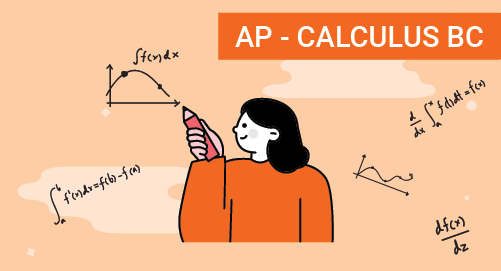#### Need Help?

Get in touch with usAP

# AP Calculus BC

Calculus BC

The AP Calculus exam, administered by the College Board, is a standardized test designed for high school students. Advanced Placement (AP) Calculus serves as an introductory college-level course in calculus. The exam evaluates students' comprehension and application of fundamental calculus concepts, including limits, derivatives, integrals, Sequence series and Parametric equations. Through problem-solving and mathematical reasoning, students demonstrate their understanding of calculus techniques and their ability to analyze and solve real-world problems. The exam covers topics such as rates of change, optimization, differential equations, and applications of integration.

## Know more about AP Calculus BC

AP Calculus BC is an Advanced Placement course and exam offered by the College Board. It is an extension of AP Calculus AB and covers additional topics in calculus. Here are some short details about AP Calculus BC:

###### Course Overview:

AP Calculus BC covers all the topics included in AP Calculus AB, as well as additional advanced calculus concepts.

It is designed for students who have a strong interest in mathematics and a solid foundation in pre-calculus and calculus AB.

Key Concepts:

• Limits and Continuity

• Derivatives and their applications

• Integrals and their applications

• Infinite series and sequences

• Parametric, polar, and vector functions

• Differential equations

###### Exam Details:

The AP Calculus BC exam assesses students' understanding of both AB and BC calculus concepts and their problem-solving skills.

• It consists of two main sections: multiple-choice questions and free-response questions.

• The multiple-choice section contains around 45 questions.

• The free-response section includes six questions.

## Test Pattern

S No Test Section Number of Questions Time Allotted
1 Multiple Choice 45 105 minutes
2 Free Response 6 90 minutes

## What you'll learn

• 1

Limits and continuity: Understanding limits conceptually and algebraically, Evaluating limits graphically and numerically, Continuity and the Intermediate Value Theorem

• 2

Differentiation: Definition and Basic Derivative Rules, Derivatives of polynomial, exponential, logarithmic, and trigonometric functions

• 3

Differentiation: Chain rule and implicit differentiation, inverse trigonometric, Higher-order derivatives

• 4

Contexual applications of differentiation: Related rate, rate of change, linearization, L’Hospital’s Rule

• 5

Analytical Applications of Differentiation: Mean value theorem, Extreme value theorem, Concavity, Optimization, Curve sketching and analysis of functions

• 6

Integration and Accumulation of Change: Areas with Reimann sum, Definite integrals and the Fundamental Theorem of Calculus, Techniques of integration, including substitution and integration by parts

• 7

Differential equations: Modeling and verifying solutions, Slope fields and separation of variables, Exponential models, Euler's method

• 8

Applications of Integration: Accumulation functions and definite integrals, Finding the Area between curves, Finding volumes using different techniques

• 9

Parametric Equations, Polar Coordinates, and Vector-Valued Functions: Parametric equations and their graphs, Vector Valued Functions and their derivatives, Polar coordinates and polar graphs

• 10

Infinite Sequences and Series: Convergence and divergence of sequences, Tests for convergence series, Taylor Polynomial Approximations, Lagrange Error Bound, Taylor or Maclaurin Series

## Skills you'll learnIdentify an appropriate mathematical rule or procedure based on the classification of a given expressionExplain how an approximated value relates to the actual valueIdentify mathematical information from graphical, numerical, analytical, and/or verbal representationsDescribe the relationships among different representations of functions and their derivativesConfirm whether hypotheses or conditions of a selected definition, theorem, or test have been satisfiedExplain the meaning of mathematical solutions in contextIdentify an appropriate mathematical definition, theorem, or test to applyConfirm that solutions are accurate and appropriateUse precise mathematical language and appropriate units of measure and symbols